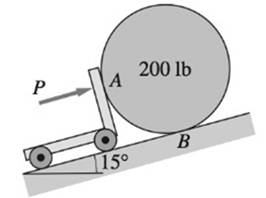# The 200-lb homogenous cylinder of radius R is pushed up the incline by a cart. Determine the smallest force P that the cart must apply to the cylinder in order to initiate motion. The coefficients of static friction are 0.3 at A and 0.12 at B . Neglect the weight of the cart.### International Edition---engineerin...

4th Edition
Andrew Pytel And Jaan Kiusalaas
Publisher: CENGAGE L
ISBN: 9781305501607### International Edition---engineerin...

4th Edition
Andrew Pytel And Jaan Kiusalaas
Publisher: CENGAGE L
ISBN: 9781305501607

#### Solutions

Chapter
Section
Chapter 7, Problem 7.9P
Textbook Problem

## The 200-lb homogenous cylinder of radius R is pushed up the incline by a cart. Determine the smallest force P that the cart must apply to the cylinder in order to initiate motion. The coefficients of static friction are 0.3 at A and 0.12 at B. Neglect the weight of the cart.Expert Solution
To determine

Smallest force 'P' that cart must apply to the cylinder.

### Explanation of Solution

Given information:

The weight of the cylinder is 200lb.

Co-efficient of static friction are 0.3 at point A and 0.12 at point B.

For impending sliding, the friction force equals its limiting value.

F=Fmax=μsN

μs - Co-efficient of static friction

Steps to follow in the equilibrium analysis of a body are:

1. Draw the free body diagram.

2. Write the equilibrium equations.

3. Solve the equations for the unknowns.

Calculation:

FBD of entire system

Assume sliding at point B.

Assume NA,NB as the reaction force at point A and B.

Write equilibrium equation in x direction.

Fx=0

NA0.12NB200sin15°=0

Write equilibrium equation in y direction.

Fy=0

NBFA200cos15°=0

For the equilibrium of above section, the bending moment about point O is equal to zero.

MO=0

FA0.12NB=0

Solve above equations simultaneously.

FA=26

### Want to see the full answer?

Check out a sample textbook solution.See solution

### Want to see this answer and more?

Bartleby provides explanations to thousands of textbook problems written by our experts, many with advanced degrees!

See solution